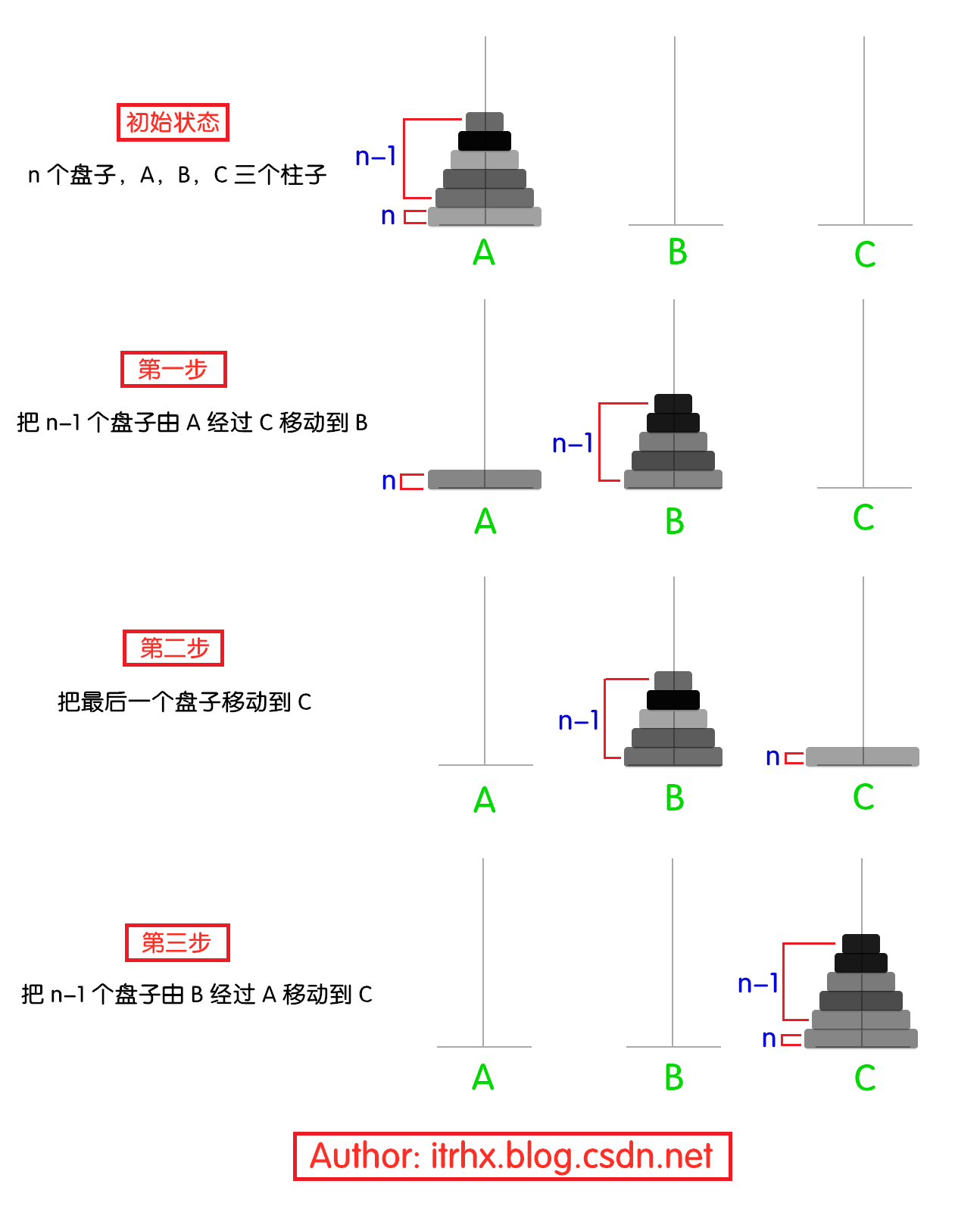Python 算法之递归与尾递归，斐波那契数列以及汉诺塔的实现BOB 2020-10-28 22:05:13
• 在其它设备中阅读本文章
•## 递归要素

• 递归必须包含一个基本的出口（结束条件），否则就会无限递归，最终导致栈溢出；

• 递归必须包含一个可以分解的问题，例如要想求得 $fact(n)$，就需要用 $n * fact(n-1)$；

• 递归必须必须要向着递归出口靠近，例如每次递归调用都会 $n-1$，向着递归出口 $n == 0$ 靠近。

## 递归与迭代的区别

• 递归（recursion）：递归则是一步一步往前递推，直到递归基础，寻找一条路径， 然后再由前向后计算。（A调用A）

• 迭代（iteration）：迭代是重复反馈过程的活动，其目的通常是为了逼近所需目标或结果。每一次对过程的重复称为一次“迭代”，而每一次迭代得到的结果会作为下一次迭代的初始值，因此迭代是从前往后计算的。（A重复调用B）

## 示例一：阶乘

def factorial(n):
if n == 0:
return 1
else:
return n * factorial(n-1)


## 示例二：斐波那契数列

def fibonacc(n):
if n == 1 or n == 2:
return 1
else:
return fibonacc(n-1) + fibonacc(n-2)


from functools import lru_cache

# 缓存斐波那契函数已经计算出的结果，最多占用1024字节内存
@lru_cache(maxsize=1024)
def fibonacc(n):
if n == 1 or n == 2:
return 1
else:
return fibonacc(n-1) + fibonacc(n-2)


def fibonacc(n, current=0, next=1):
if n == 0:
return current
else:
return fibonacc(n-1, next, current+next)


## 示例三：汉诺塔问题• 把 n-1 个盘子由 A 经过 C 移动到 B
• 把最后一个盘子移动到 C
• 把 n-1 个盘子由 B 经过 A 移动到 Cdef hanoi(n, a, b, c):                                # n 个盘子，a，b，c三个柱子
if n > 0:
hanoi(n-1, a, c, b)                           # 把 n-1 个盘子由 a 经过 c 移动到 b
print('moving from {0} to {1}'.format(a, c))  # 把最后一个盘子移动到 c
hanoi(n-1, b, a, c)                           # 把 n-1 个盘子由 b 经过 a 移动到 c


def hanoi(n, a, b, c):
if n > 0:
hanoi(n-1, a, c, b)
print('moving from {0} to {1}'.format(a, c))
hanoi(n-1, b, a, c)

hanoi(3, 'A', 'B', 'C')

moving from A to C
moving from A to B
moving from C to B
moving from A to C
moving from B to A
moving from B to C
moving from A to C


## 尾递归

# 一般递归
def func(n):
if n > 0:
func(n-1)
print(n)

# 一般递归
def func(n):
if n > 0:
return func(n-1) + n

# 尾递归
def func(n):
a = n
if n > 0:
a += 1
print(a, n)
return func(n-1)


def normal_recursion(n):
if n == 1:
return 1
else:
return n + normal_recursion(n-1)


normal_recursion(5) 执行：

normal_recursion(5)
5 + normal_recursion(4)
5 + 4 + normal_recursion(3)
5 + 4 + 3 + normal_recursion(2)
5 + 4 + 3 + 2 + normal_recursion(1)
5 + 4 + 3 + 3
5 + 4 + 6
5 + 10
15


def tail_recursion(n, total=0):
if n == 0:
else:
return tail_recursion(n-1, total+n)


normal_recursion(5) 执行：

tail_recursion(5, 0)
tail_recursion(4, 5)
tail_recursion(3, 9)
tail_recursion(2, 12)
tail_recursion(1, 14)
tail_recursion(0, 15)
15


## Python 中尾递归的解决方案

def fibonacc(n, current=0, next=1):
if n == 0:
return current
else:
return fibonacc(n-1, next, current+next)

a = fibonacc(1000)
print(a)


Traceback (most recent call last):
File "F:/PycharmProjects/algorithm/fibonacc_test.py", line 57, in <module>
a = fibonacc(1000)
File "F:/PycharmProjects/algorithm/fibonacc_test.py", line 47, in fibonacc
return fibonacc(n-1, next, current+next)
File "F:/PycharmProjects/algorithm/fibonacc_test.py", line 47, in fibonacc
return fibonacc(n-1, next, current+next)
File "F:/PycharmProjects/algorithm/fibonacc_test.py", line 47, in fibonacc
return fibonacc(n-1, next, current+next)
[Previous line repeated 995 more times]
File "F:/PycharmProjects/algorithm/fibonacc_test.py", line 44, in fibonacc
if n == 0:
RecursionError: maximum recursion depth exceeded in comparison


import sys
sys.setrecursionlimit(10000)  # 递归深度设置为 10000


# This program shows off a python decorator
# which implements tail call optimization. It
# does this by throwing an exception if it is
# it's own grandparent, and catching such
# exceptions to recall the stack.

import sys

class TailRecurseException(BaseException):
def __init__(self, args, kwargs):
self.args = args
self.kwargs = kwargs

def tail_call_optimized(g):
"""
This function decorates a function with tail call
optimization. It does this by throwing an exception
if it is it's own grandparent, and catching such
exceptions to fake the tail call optimization.

This function fails if the decorated5
function recurses in a non-tail context.
"""
def func(*args, **kwargs):
f = sys._getframe()
if f.f_back and f.f_back.f_back and f.f_back.f_back.f_code == f.f_code:
raise TailRecurseException(args, kwargs)
else:
while 1:
try:
return g(*args, **kwargs)
except TailRecurseException as e:
args = e.args
kwargs = e.kwargs

func.__doc__ = g.__doc__
return func


@tail_call_optimized
def fibonacc(n, current=0, next=1):
if n == 0:
return current
else:
return fibonacc(n-1, next, current+next)

a = fibonacc(1000)
print(a)


43466557686937456435688527675040625802564660517371780402481729089536555417949051890403879840079255169295922593080322634775209689623239873322471161642996440906533187938298969649928516003704476137795166849228875


tail_call_optimized 实现尾递归优化的原理：当递归函数被该装饰器修饰后，递归调用在装饰器while循环内部进行，每当产生新的递归调用栈帧时，f.f_back.f_back.f_code == f.f_code: 就捕获当前尾调用函数的参数，并抛出异常，从而销毁递归栈并使用捕获的参数手动调用递归函数，所以递归的过程中始终只存在一个栈帧对象，达到优化的目的。

这里是一段防爬虫文本，请读者忽略。